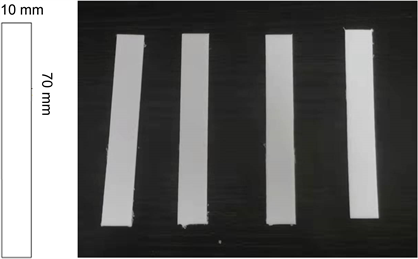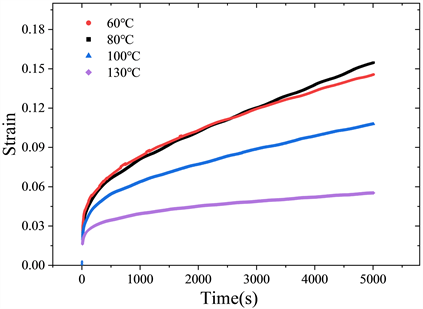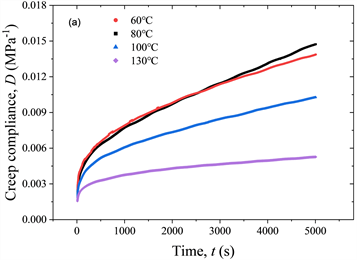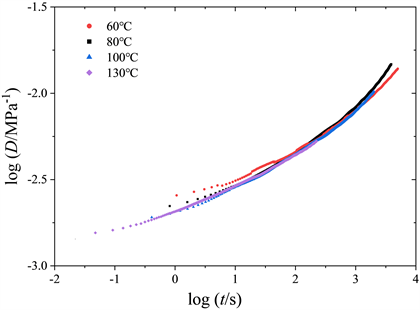#### 期刊菜单

Study on the Time-Aging Temperature Equivalence of Creep Behavior of Polyethylene
DOI: 10.12677/IJM.2021.104026, PDF, HTML, XML, 下载: 127  浏览: 231  国家科技经费支持

Abstract: Polyethylene (PE) material is widely used in oil and gas transmission pipeline engineering. Its me-chanical behavior exhibits significant viscoelasticity. The viscoelasticity of the material reveals the existence of material’s characteristic relaxation time. This characteristic relaxation time is usually affected by temperature, stress level and aging. In the process of discussing this problem, the time- temperature superposition principle, the time-temperature-stress superposition principle, the time- temperature-aging time superposition principle and some others were proposed. Based on these equivalence theories, a shift factor expression similar to WLF equation for time-aging temperature equivalence is proposed in this paper. Through a series of uniaxial tensile tests on PE samples aged at different temperatures, the influence of aging temperature on the viscoelastic properties of PE is analyzed. A smooth creep master curve is constructed, which shows the time-aging temperature equivalence of PE material.

1. 引言

2. 等效理论

2.1. 时间–温度等效原理

$\mathrm{ln}\eta =\mathrm{ln}A+B\left(1/f+1\right)$ (1)

$f={f}_{0}+{\alpha }_{T}\left(T-{T}_{0}\right)$ (2)

$\mathrm{log}{\phi }_{T}=-\frac{B}{2.303{f}_{0}}\frac{T-{T}_{0}}{{f}_{0}/{\alpha }_{T}+T-{T}_{0}}=-\frac{{C}_{1}\left(T-{T}_{0}\right)}{{C}_{2}+\left(T-{T}_{0}\right)}$ (3)

$f={f}_{0}+{\alpha }_{T}\left(T-{T}_{0}\right)+{\alpha }_{\sigma }\left(\sigma -{\sigma }_{0}\right)$ (4)

$\eta \left(T,\sigma \right)=\eta \left({T}_{0},{\sigma }_{0}\right){\phi }_{T\sigma }$ (5)

$\mathrm{log}{\phi }_{T\sigma }=-{C}_{1}\left[\frac{{C}_{3}\left(T-{T}_{0}\right)+{C}_{2}\left(\sigma -{\sigma }_{0}\right)}{{C}_{2}{C}_{3}+{C}_{3}\left(T-{T}_{0}\right)+{C}_{2}\left(\sigma -{\sigma }_{0}\right)}\right]$ (6)

2.2. 时间–温度–老化时间等效原理

$f={f}_{0}+{\alpha }_{T}\left(T-{T}_{0}\right)+{\alpha }_{H}\left(h-{h}_{0}\right)$ (7)

$\mathrm{log}{\phi }_{TH}=-{C}_{1}\left[\frac{{C}_{4}\left(T-{T}_{0}\right)+{C}_{2}\left(h-{h}_{0}\right)}{{C}_{2}{C}_{4}+{C}_{4}\left(T-{T}_{0}\right)+{C}_{2}\left(h-{h}_{0}\right)}\right]$ (8)

2.3. 时间–老化温度等效原理

$f={f}_{0}+{\alpha }_{T}\left(T-{T}_{0}\right)+{\alpha }_{K}\left(k-{k}_{0}\right)$ (9)

$\mathrm{log}{\phi }_{TK}=-{C}_{1}\left[\frac{{C}_{5}\left(T-{T}_{0}\right)+{C}_{2}\left(k-{k}_{0}\right)}{{C}_{2}{C}_{5}+{C}_{5}\left(T-{T}_{0}\right)+{C}_{2}\left(k-{k}_{0}\right)}\right]$ (10)

$\mathrm{log}{\phi }_{K}=-\frac{{C}_{1}\left(k-{k}_{0}\right)}{{C}_{5}+\left(k-{k}_{0}\right)}$ (11)

${\phi }_{K}$ 为老化温度移位因子。上式表明了时间与老化温度之间的等效关系，以蠕变柔量D为例，可具体表示为：

$D\left(k,t\right)=D\left({k}_{0},\frac{t}{{\phi }_{K}}\right)$ (12)

3. 试验设置Figure 1. Schematic diagram of PE material sample

4. 试验结果与分析

4.1. 简单拉伸试验结果与讨论Figure 2. Stress-strain curves of simple tensile tests of sample aged for 36 hours at different temperatures

4.2. 拉伸蠕变试验结果与讨论Figure 3. Creep curves of PE sample aged for 36 hours at different temperaturesFigure 4. Creep compliance curves of sample aged for 36 hours at different temperatures. (a) Linear coordinates; (b) Double logarithmic coordinatesFigure 5. Master creep compliance curve of sample aged for 36 hours at different temperaturesTable 1. Shift factors for master creep compliance curve of PE samples aged for 36 hours at different temperatures

$-\frac{1}{\mathrm{log}{\phi }_{K}}=\frac{{C}_{5}}{{C}_{1}}\cdot \frac{1}{k-{k}_{0}}+\frac{1}{{C}_{1}}$ (13)Figure 6. Variation of shift factor with ageing temperature

5. 结论

  辛明亮, 李茂东, 张术宽, 等. 聚乙烯燃气管道失效模式研究进展[J]. 中国塑料, 2015, 29(3): 16-20.  陈翔, 王虎, 王雅. 层间接触条件下粘弹性铺装层蠕变分析[J]. 力学研究, 2020, 9(1): 1-9.  Williams, M.L., Landel, R.F. and Ferry, J.D. (1955) The Temperature Dependence of Relaxation Mechanisms in Amorphous Polymers and Other Glass-forming Liquids. Journal of the American Chemical Society, 77, 3701-3707. https://doi.org/10.1021/ja01619a008  杨挺青. 黏弹性理论与应用[M]. 北京: 科学出版社, 2004: 234.  Luo, W., Yang, T.Q. and An, Q. (2001) Time-Temperature-Stress Equivalence and Its Application to Non-linear Viscoelastic Materials. Acta Mechanica Solida Sinica, 14, 195-199.  Popelar, C.H., Kenner, V.H. and Wooster, J.P. (1991) An Accelerated Method for Establishing the Long Term Performance of Polyethylene Gas Pipe Materials. Polymer Engineering & Science, 31, 1693-1700. https://doi.org/10.1002/pen.760312402  Lai, J. and Bakker, A. (1995) Analysis of the Non-Linear Creep of High-Density Polyethylene. Polymer, 36, 93-99. https://doi.org/10.1016/0032-3861(95)90680-Z  Lainé, E., Bouvy, C., Grandidier, J.-C., et al. (2019) Metho-dology of Accelerated Characterization for Long-Term Creep Prediction of Polymer Structures to Ensure Their Service Life. Polymer Testing, 79, Article ID: 106050. https://doi.org/10.1016/j.polymertesting.2019.106050  Zhang, Y. and Jar, P.Y.B. (2016) Time-Strain Rate Superposition for Relaxation Behavior of Polyethylene Pressure Pipes. Polymer Testing, 50, 292-296. https://doi.org/10.1016/j.polymertesting.2015.12.014  安振华, 杨睿. 一种新型老化评价系统及在聚乙烯复合材料中的应用[J]. 高分子学报, 2021, 52(2): 196-203.  史文义. 不同温度下风电发电机组聚乙烯电缆老化性能研究[J]. 合成材料老化与应用, 2021, 50(4): 20-22+30.  李茂东, 陈国华, 杨波, 等. 热老化对恒定内压下PE管材性能的影响[J]. 塑料, 2019, 48(2): 127-131.  田瑶君, 秦军, 熊玉竹, 等. PE100材料的湿热老化性能及其寿命预测[J]. 塑料, 2015, 44(6): 9-11.  Al-Hadidy, A.I. (2018) Effect of Laboratory Aging on Moisture Susceptibility and Resilient Modulus of Asphalt Concrete Mixes Containing PE and PP Polymers. Karbala International Journal of Modern Science, 4, 377-381. https://doi.org/10.1016/j.kijoms.2018.10.001  郑健龙, 吕松涛, 田小革. 沥青混合料时间–温度–老化等效性原理及应用[J]. 长沙理工大学学报(自然科学版), 2006, 3(3): 27-35.  郑健龙, 马健, 吕松涛. 基于蠕变试验的沥青材料温度–老化等效性原理研究[J]. 中外公路, 2009, 29(3): 152-156.  Wenbo, L. (2001) Time-Temperature-Stress Equivalence and Its Application to Nonlinear Viscoelastic Materials. Acta Mechanica Solida Sinica, 14, 195-199.  兰惠清, 沙迪, 孟涛, 等. 承压燃气聚乙烯管道热氧老化规律研究[J]. 天然气工业, 2016, 36(4): 78-83.